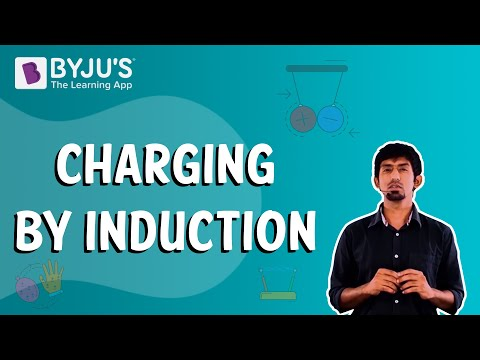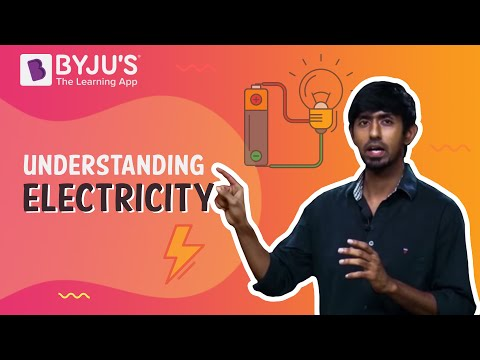# Basic Properties Electrical Charge Questions

## Electric Charge

Electric charge can be defined as the physical property of matter that makes it experience a force when kept in an electric or magnetic field.

## Properties of Electric Charges

Consider the electric charges to be really small, known as the point charge in order to understand at the properties of electric charge

Electric charges are scalar in nature but it is important to note that charges can be point charges, and still are positive and negative in nature.

If inside a conductor there are ‘n’ number of charges present, the algebraic sum of the individual charges will be the total charge present in it. This is the additive property of electric charges.

Q = q1+q2+q3+……qn

2. Conservation of Charges

The conservation of charges means that the charges are neither created nor destroyed. They can be transferred from one body to another. The charges are always conserved in an Isolated system.

3. Quantization of Charge

Electric charges are defined as the integral multiple of the charge present on them, hence, in any system the charges will be, Q = ne

### Some other properties of electric charges are –

• Charge is a scalar quantity.
• Charge transfer from one body to another which means they are movable.
• Like charges repel each other and unlike charges attract each other.
• Charge is always linked with mass.

### Important Questions on Electric Charges

1) Which of the following is not the property of charge?

b) A charge is self-destructive

c) Quantization of charge

d) Charge is conserved

Correct Option: (b)

Explanation: Electric charge holds the quantization property, conservation of charge, also it cannot be destroyed therefore it is not self-destructive.

2) Earth is the source of_____

a) Zero charge

b) Positive charge

c) Negative charge

d) An infinite positive and negative charge

Correct Option: (d)

Explanation: If a negatively or positively charged body is connected to the ground, all of its charges will go to earth. Therefore, it is clear that earth is an infinite source of positive and negative charges.

3) Which among the following should be the perfect shape of a conductor that can hold a charge for a long time?

a) Cubical

b) Spherical

c) Sharp-edged

d) Conical

Correct Option: (b)

Explanation: Over the entire surface of the sphere there exists a uniform charge distribution. Since it doesn’t have any sharp edges, hence very less chances of charge accumulation at edges. This results in very less chances of discharge of stored charge.

4) Which among the following is the safest place during lightning?

a) Under a tree

b) High wall

c) House with lightning arrester

d) Under a light post

Correct Option: (c)

Explanation: Lightning arrester captures lightning and allows a safe path of electricity to ground. Hence, a safe place will be inside a house that has a lightning arrester on the top of it.

5) What will be the total charge stored in the system, if +q, +2q, +3q, +4q, …… (up to +20q) charges are positioned at coordinates (0,0) , (1,0) ,(2,0) , ….. (Up to 20)?

a) +20q

b) +420q

c) +210q

d) +190q

Correct Option: (c)

Explanation: The total charge will be

= (1+2+3+4+…. +20)*q = [(20*21)/2]*q = 210q.

But the result will be different if the polarities of the charges are different. We will add the positive charges and the negative charges separately.

6) More charge will be carried by a solid sphere rather than a sphere which is hollow but of the same radius. Is it true or false?

a) True

b) False

Correct Option: (b)

Explanation: As both the spheres have the same radius and same surface area, they will carry the same amount of charge because the charge is distributed only on the outer surface of a body.

7) For which of the following options, this statement is true – A charged conductor has its charge only on its outer surface.

a) For hollow conductors

b) For those conductors which don’t have sharp edges

c) For all conductors

d) Only for spherical conductors

Correct Option: (c)

Explanation: Regardless of the shape and size of the conductor, the charge remains on the outer surface, for both solid and hollow conductors.

8) What is the SI unit of surface charge density?

a) C

b) C/m2

c) C/m

d) C/m3

Correct Option: (b)

Explanation: The amount of charge that is stored in the unit surface area of a conductor is known as the surface charge density.

So, it’s unit will be = (the unit of the charge/the unit of area).

Since, the SI unit of charge is Coulomb and the unit of area is m2. Therefore, the essential unit will be C/m2.

9) The number of electrons flowing in one minute through a conductor carrying 1 ampere current will be?

a) 5.2*1020

b) 4.2*1020

c) 3.7*1019

d) 3.7*1020

Correct Option: (d)

Explanation: The number of electron flow per minute

= (1/1.602∗10−19) *60 =3.7*1020.

Since, 1 Ampere means 1 Coulomb per second and the number of electrons flowing per second is (1/1.602∗10−19).

10) A lightning arrester should possess either of the following properties.

a) Discontinuity

b) Poor conductivity

c) Low melting point

d) Needle end

Correct Option: (d)

Explanation: Needle end has the property to arrest lightning easily. Also, devices must deliver a continuous path to electricity so that current passes to ground. It must possess a higher melting point to avoid the melting of the needle due to the heat generated during carrying lightning current.

### Practice Questions

1. Explain the distribution of electric charges within the atom.
2. Define and explain Coulomb’s law.
3. What are the basic properties of electric charges?
4. Give a brief description about different methods of charging.
5. Define 1 Ampere.

 Related Articles

## Suggested VideosStay tuned to BYJU’S and Fall in Love with Learning!